# MCQs on Electric Circuits

##### Page 26 of 63. Go to page 1 2 3 4 5 6 7 8 9 10 11 12 13 14 15 16 17 18 19 20 21 22 23 24 25 26 27 28 29 30 31 32 33 34 35 36 37 38 39 40 41 42 43 44 45 46 47 48 49 50 51 52 53 54 55 56 57 58 59 60 61 62 63
01․ The resistance of 100 W, 200 V lamp is
200 Ω.
400 Ω.
800 Ω.
1600 Ω.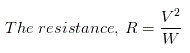Where, V is supply voltage and W is wattage rating of the lamp. Here, wattage of the lamp W = 100 W, and supply voltage V = 200 Volts.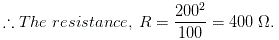02․ Purpose of using capacitor is / are
increase p.f of inductive load circuit.
to do phase split in AC 1 phase motor.
effect DC filter in electronic circuit.
all of these.

Purposes of using capacitors are 1. Increasing p.f. of inductive load circuit. 2. To do phase split in AC 1 phase motor. 3. Effect DC filter in electronic circuit. 4. Also helps in tuning in radio and TV sets.

03․ The unit of capacitance is
Coulomb.
Henry.
Weber.

A farad (F) is the SI derived unit of capacitance. A capacitor of one farad produces a potential difference of one volt between its plates when it stores an electric charge of one coulomb.

04․ What will be the capacitance when distance between the 2 plates of a condenser of capacitance 8 micro farad is reduced from 10 mm to 4 mm?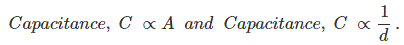Where, A is common area of conductor plates and d is the distance between two conductor plates.

05․ The capacitance of a conductor is varying from 2 microfarad to zero in 1 sec linearly if the voltage applied to it is 6 V the energy stored in 0.5 sec in the condenser is
55.1 µ joules.
18 µ joules.
10 µ joules.
20 µ joules.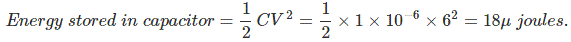06․ A 50 μF capacitor is charged to retain 10 MJ of energy by a constant charging current of 1 A. Determine the voltages across the capacitor?
30 V.
20 V.
50 V.
60 V.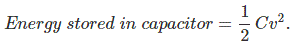Where v being the voltage developed across the capacitor of capacitance(C).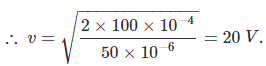07․ A p.d. of 300 V is applied across series combination of 3 μF and 9 μF capacitors. The charge on each capacitor is ___________________ μC.
675.
3600.
240.
7.5.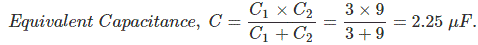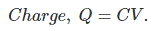08․ A capacitor that stores charge of 0.5 C at 10 V has a capacitance of _____________ farad.
5.
0.05.
10.
20.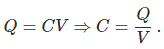09․ A capacitor has a capacitance of 6 μF. Calculate the stored energy in it if a DC voltage of 100 V, is applied across it?
3 × 102 joules.
2.5 × 102 joules.
6 × 102 joules.
4 × 102 joules.10․ Ceq of two capacitors connected in series is given by
C1C2.
C1 = C2.
C1 C2 / (C1 + C2).
C1 + C2.

Series combination of capacitors is same as parallel combination of resistance.

<<<2425262728>>>